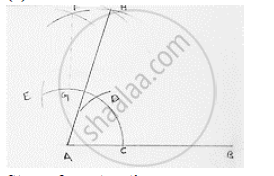# Construct the Angle of the Measurement: 1 . 75° - Mathematics

Construct the angle of the measurement:

1 . 75°

#### SolutionSteps of construction:
1. Draw a line segment AB.
2. With center A any radius, draw an arc which intersect AB at C.
3. With center C and same radius, draw an arc which intersects previous arc at D.
4. With center D and same radius, draw an arc which interest are in (2) at E
5. With centers E and D and radius more than 1/2ED draw arcs intersecting each other at F.
6. Join FA which intersects arc in (2) at G

7. With centers G and D, and radius more than 1/2 GD draw arcs intersecting each other at H.
8. Join HA

∴ ∠HAB = 75°

Concept: Basic Constructions
Is there an error in this question or solution?

#### APPEARS IN

RD Sharma Mathematics for Class 9
Chapter 16 Constructions
Exercise 16.2 | Q 11.2 | Page 9

Share